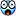# Bouyant force

I have a little confusion regarding bouyancy. Suppose two objects fully immersed in liquid having equal volumes, one close to surface but other at greater depth. As both displace equal volumes of water therefore they should be facing equal bouyant forces. But as the object which is at greater depth should be facing more pressure and hence more force. Is it? Or they are facing equal forces but due to difference in densities they are at different heights? Please help.

Chestermiller
Mentor
The thing that determines the buoyant force is the pressure variation from bottom to top of the object, and not the absolute pressure on the object.

Chet

•Rayquesto
Dale
Mentor
2021 Award
As Chestermiller says the pressure on the bottom is indeed greater, but so is the pressure on the top. The buoyant force is the difference between the two, which is the same at either depth.

As Chestermiller says the pressure on the bottom is indeed greater, but so is the pressure on the top. The buoyant force is the difference between the two, which is the same at either depth.
Okay but the object at greater depth is due to its greater density.

BruceW
Homework Helper
eh? yes. the more dense object will usually end up deeper. But this is not necessarily true, since you might fix it's height with a chain. And then you could measure the buoyant force through the chain.

eh? yes. the more dense object will usually end up deeper. But this is not necessarily true, since you might fix it's height with a chain. And then you could measure the buoyant force through the chain.
Thanks

BruceW
Homework Helper
oh, and you'd need to account for the weight of the object, if you were trying to measure buoyant force by using the chain.

Chestermiller
Mentor
Okay but the object at greater depth is due to its greater density.
The buoyant force is determined by the density of the fluid that the object is immersed in, not the density of the object.

Chet

•Rayquesto
RonL
Gold Member
Last edited by a moderator:
The buoyant force is determined by the density of the fluid that the object is immersed in, not the density of the object.

Chet
I am talking about the depths achieved by two objects that displace equal volumes of fluid.

Dale
Mentor
2021 Award
Okay but the object at greater depth is due to its greater density.
No. Not necessarily. If the fluid is incompressible (what is usually implied by the word "liquid") then the density is constant regardless of depth/pressure.

You seem to be thinking of a gas, not a liquid.

oh, and you'd need to account for the weight of the object, if you were trying to measure buoyant force by using the chain.
I have another mathematical confusion. As the Bouyant force is equal to weight of water displaced which is equal to weight of object and both have same volumes, therefore g and Volume are canceled out which leaves: density of water= density of object, which is not possible. Mathematically,
ρ(water)V(fluid)g=ρ(object)V(object)g
ρ(water)=ρ(object)

Doug Huffman
Gold Member
I learned the hard way that water has a small but significant bulk modulus of elasticity/compressibility, about 1/3000 as I recall many years later.

Dale
Mentor
2021 Award
the Bouyant force is equal to weight of water displaced which is equal to weight of object
For a submerged object this is only true if the object is neutrally buoyant.

russ_watters
Mentor
I am talking about the depths achieved by two objects that displace equal volumes of fluid.
Well, for air (for example), density increases with "depth" in the atmosphere, so yes, pressure increases with the density of the fluid, as the object sinks. But if you're trying to get back to pressure as the driver for buoyancy change in general, no, it isn't correct. Higher density and therefore buoyancy can also be compared between different fluids, regardless of the pressure.

BruceW
Homework Helper
I have another mathematical confusion. As the Bouyant force is equal to weight of water displaced which is equal to weight of object and both have same volumes, therefore g and Volume are canceled out which leaves: density of water= density of object, which is not possible. Mathematically,
ρ(water)V(fluid)g=ρ(object)V(object)g
ρ(water)=ρ(object)
yeah, DaleSpam has the right idea. The Buoyant force on the object equals the weight of water displaced, not the weight of the object. These things are only equal in the special case when the (average) density of the object is the same as the density of the water, i.e. neutrally buoyant.

edit: and Chestermiller mentioned this earlier.

For a submerged object this is only true if the object is neutrally buoyant.
Thanks for the clarification.

RonL
Gold Member
I learned the hard way that water has a small but significant bulk modulus of elasticity/compressibility, about 1/3000 as I recall many years later.
Were you water pressure testing a tank ?

Doug Huffman
Gold Member
Yes, a hydrostatic test IIRC as 3000 psi of a 600 psi NOP steam drum. The test relief valve apparently lifted inappropriately (I test director directly supervised the operators and primary test gauge) and the only physical evidence that I had was the volume of water relieved and the relief valve that retested properly.

RonL
Gold Member
Yes, a hydrostatic test IIRC as 3000 psi of a 600 psi NOP steam drum. The test relief valve apparently lifted inappropriately (I test director directly supervised the operators and primary test gauge) and the only physical evidence that I had was the volume of water relieved and the relief valve that retested properly.
I had the similar experience at a much smaller and lower pressure scale, what I thought would be a simple unloading of pressure produced a bit of surprise (no damage or injury) I'm always very Leary of pressure and spin speed